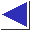# comp.lang.c FAQ list ·Question 14.11

Q: What's a good way to implement complex numbers in C?

A: It is straightforward to define a simple structure and some arithmetic functions to manipulate them. C99 supports complex as a standard type. [footnote] Here is a tiny example, to give you a feel for it:

```typedef struct {
double real;
double imag;
} complex;

#define Real(c) (c).real
#define Imag(c) (c).imag

complex cpx_make(double real, double imag)
{
complex ret;
ret.real = real;
ret.imag = imag;
return ret;
}

{
return cpx_make(Real(a) + Real(b), Imag(a) + Imag(b));
}
```
You can use these routines with code like
```	complex a = cpx_make(1, 2);
complex b = cpx_make(3, 4);
```	complex c = cpx_add(cpx_make(1, 2), cpx_make(3, 4));